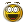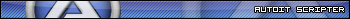Recommended Posts

```\$ok=MouseGetPos()
\$ok=\$xt

\$hDC = _WinAPI_GetWindowDC(0)
\$o_Orig = _WinAPI_SelectObject(\$hDC, \$hPen)
\$hPen = _WinAPI_CreatePen(\$PS_SOLID, \$iWidth, 0xFFFFFF)

While \$ok<\$disty+\$xt
\$ok= \$ok+5
\$o = \$ok-\$xt
\$y=MouseGetPos()
_WinAPI_DrawLine(\$hDC,\$ok ,\$y-((\$fsiny*\$o/\$fcosy)-(9.8*\$o*\$o/(2*10367.3435771*\$fcosy*\$fcosy))), \$ok, \$y-((\$fsiny*\$o/\$fcosy)-(9.8 * \$o*\$o/(2*10367.3435771*\$fcosy*\$fcosy)))-3)

Wend```

So I have this function , it doesn't really matter, it will just draw a graph . But since the screen refreshes constantly, I can't see the lines that I'm drawing for more than one second, therfore I can't see the graph of the function at all.

How can I make it stay? Other resources than _WinAi_DrawLine ? I need it to be right on the screen . Not in a GUI so I guess I can't use GDI.

Share on other sites

Can you show a working code?...

SaludosDanysys.comAutoIt...

Share on other sites
```#include <Math.au3>
#include <MsgBoxConstants.au3>
#include <WinAPI.au3>
#include <WindowsConstants.au3>

\$x=MouseGetPos()
\$dist=444
\$fDegree = _Degree(ASin(\$dist*9.8/10367.3435771))
\$unghi=(180-\$fDegree)/2
\$unghi2=\$fDegree/2
\$fcos =Abs(Cos(\$unghi*3.14159265/180))
\$fsin =Abs(sin(\$unghi*3.14159265/180))
deseneaza(\$fcos, \$fsin, 20, 2, 0x0000FF, \$x, \$dist)

Func deseneaza(\$fcosy, \$fsiny, \$iLength, \$iWidth, \$iColor, \$xt , \$disty)
Local \$hDC, \$hPen, \$o_Orig

\$hDC = _WinAPI_GetWindowDC(0)
\$hPen = _WinAPI_CreatePen(\$PS_SOLID, \$iWidth, \$iColor)
\$o_Orig = _WinAPI_SelectObject(\$hDC, \$hPen)

_WinAPI_DrawLine(\$hDC, MouseGetPos() , MouseGetPos(), MouseGetPos()+138*\$fcosy, MouseGetPos()-138*\$fsiny)

; clear resources
_WinAPI_SelectObject(\$hDC, \$o_Orig)
_WinAPI_DeleteObject(\$hPen)
_WinAPI_ReleaseDC(0, \$hDC)

\$ok=MouseGetPos()
\$ok=\$xt

\$hDC = _WinAPI_GetWindowDC(0)
\$o_Orig = _WinAPI_SelectObject(\$hDC, \$hPen)
\$hPen = _WinAPI_CreatePen(\$PS_SOLID, \$iWidth, 0xFFFFFF)

While \$ok<\$disty+\$xt
\$ok= \$ok+5
\$o = \$ok-\$xt
\$y=MouseGetPos()
_WinAPI_DrawLine(\$hDC,\$ok ,\$y-((\$fsiny*\$o/\$fcosy)-(9.8*\$o*\$o/(2*10367.3435771*\$fcosy*\$fcosy))), \$ok, \$y-((\$fsiny*\$o/\$fcosy)-(9.8 * \$o*\$o/(2*10367.3435771*\$fcosy*\$fcosy)))-3)

Wend

EndFunc```

It's really messy cause it's just a bit of the code . Anyway , try it over a flash / browser or just click one time and the graph just goes away.

Share on other sites

Store the line on variables. then draw while loop.

SaludosDanysys.comAutoIt...

Share on other sites

I don't get it? I should create an array that stores every single y? And then wouldnt it be the same anyway ?

Share on other sites

I meant this,

```#include <Math.au3>
#include <MsgBoxConstants.au3>
#include <WinAPI.au3>
#include <WindowsConstants.au3>

\$x=MouseGetPos()
\$dist=444
\$fDegree = _Degree(ASin(\$dist*9.8/10367.3435771))
\$unghi=(180-\$fDegree)/2
\$unghi2=\$fDegree/2
\$fcos =Abs(Cos(\$unghi*3.14159265/180))
\$fsin =Abs(sin(\$unghi*3.14159265/180))
deseneaza(\$fcos, \$fsin, 20, 2, 0x0000FF, \$x, \$dist)

Func deseneaza(\$fcosy, \$fsiny, \$iLength, \$iWidth, \$iColor, \$xt , \$disty)
Local \$hDC, \$hPen, \$o_Orig

\$hDC = _WinAPI_GetWindowDC(0)
\$hPen = _WinAPI_CreatePen(\$PS_SOLID, \$iWidth, \$iColor)
\$o_Orig = _WinAPI_SelectObject(\$hDC, \$hPen)

Local \$x1,\$y1,\$y2,\$x2
\$x1=MouseGetPos()
\$y1=MouseGetPos()
\$x2=MouseGetPos()+138*\$fcosy
\$y2=MouseGetPos()-138*\$fsiny

\$ok=MouseGetPos()
\$ok=\$xt

\$hDC = _WinAPI_GetWindowDC(0)
\$o_Orig = _WinAPI_SelectObject(\$hDC, \$hPen)
\$hPen = _WinAPI_CreatePen(\$PS_SOLID, \$iWidth, 0xFFFFFF)

While \$ok<\$disty+\$xt
_WinAPI_DrawLine(\$hDC, \$x1, \$y1, \$x2, \$y2)
\$ok= \$ok+5
\$o = \$ok-\$xt
\$y=MouseGetPos()
_WinAPI_DrawLine(\$hDC,\$ok ,\$y-((\$fsiny*\$o/\$fcosy)-(9.8*\$o*\$o/(2*10367.3435771*\$fcosy*\$fcosy))), \$ok, \$y-((\$fsiny*\$o/\$fcosy)-(9.8 * \$o*\$o/(2*10367.3435771*\$fcosy*\$fcosy)))-3)

Wend

; clear resources
_WinAPI_SelectObject(\$hDC, \$o_Orig)
_WinAPI_DeleteObject(\$hPen)
_WinAPI_ReleaseDC(0, \$hDC)
EndFunc```

SaludosDanysys.comAutoIt...